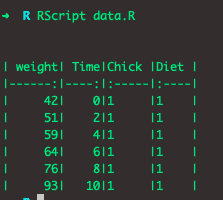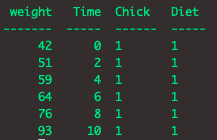# kable in R: Create tables in LaTeX, HTML, and reStructuredText

To create an Awesome HTML Table, LaTex, markdown, or simple table in R, use the knitr package. The kable() function of the knitr package is a very simple table generator, and it is simple by design. Let’s learn in detail about kable() function.

## kable in R

The kable() is a function of the knitr package, which is a straightforward table generator and is simple by design. The kable() function only generates tables for strictly rectangular data such as matrices and data frames.

The kable() method returns a single table for a single data object and returns a table that contains multiple tables if the input object is a list of data objects.

### Syntax

kable(
x,
format,
digits = getOption("digits"),
row.names = NA,
col.names = NA,
align,
caption = NULL,
label = NULL,
format.args = list(),
escape = TRUE,
...
)

### Arguments

x: It is an R Object.

format: It is a character string.

digits: It is the maximum number of digits for numeric columns.

row.names: It is a logical argument whether to include row names.

col.names: It is a character vector of column names to be used in the table.

align: It is a column alignment – a character vector consisting of “l” (left), “c” (center) and/or “r” (right).

caption: The table caption.

label: It is a table reference label.

format.args: It is a list of arguments to be passed to format() function to format table values, e.g. list(big.mark = ‘,’).

escape: It is a boolean value whether to escape special characters when producing HTML or LaTeX tables.

### Return Value

It is a character vector of the table source code.

In most cases, knitr::kable(x) takes only a data object x for getting a simple table. The format argument is automatically set according to the knitr source document format. Its possible values are pipe (tables with columns separated by pipes), simple (Pandoc’s simple tables), latex (LaTeX tables), html (HTML tables), and rst (reStructuredText tables).

### Example

Let’s take the ChickWeight dataset in this example and create a kable using the knitr::kable() function. The ChickWeight is a built-in R dataset that you can use anywhere in your program.

data <- head(ChickWeight[, 1:4])

Now, pass this data to the knitr::kable() function.

data <- head(ChickWeight[, 1:4])

print(knitr::kable(data, "pipe"))

#### Output## Generate simple table using kable() function in R

To generate a simple table using the kable() function, pass the “simple” argument.

data <- head(ChickWeight[, 1:4])

print(knitr::kable(data, "simple"))

#### OutputThe formats pipe and simple are portable. For example, they work for any output document format. Other table formats only work for specific output formats, For example, format = “latex” only works for LaTeX output documents. Using a specific table format will give you more control, at the price of sacrificing portability.

## Create an HTML table using kable() function in R

To create an HTML table using the kable() function, pass the “html” as an argument.

data <- head(ChickWeight[, 1:4])

print(knitr::kable(data, "html"))

#### Output

<table>
<tr>
<th style="text-align:right;"> weight </th>
<th style="text-align:right;"> Time </th>
<th style="text-align:left;"> Chick </th>
<th style="text-align:left;"> Diet </th>
</tr>
<tbody>
<tr>
<td style="text-align:right;"> 42 </td>
<td style="text-align:right;"> 0 </td>
<td style="text-align:left;"> 1 </td>
<td style="text-align:left;"> 1 </td>
</tr>
<tr>
<td style="text-align:right;"> 51 </td>
<td style="text-align:right;"> 2 </td>
<td style="text-align:left;"> 1 </td>
<td style="text-align:left;"> 1 </td>
</tr>
<tr>
<td style="text-align:right;"> 59 </td>
<td style="text-align:right;"> 4 </td>
<td style="text-align:left;"> 1 </td>
<td style="text-align:left;"> 1 </td>
</tr>
<tr>
<td style="text-align:right;"> 64 </td>
<td style="text-align:right;"> 6 </td>
<td style="text-align:left;"> 1 </td>
<td style="text-align:left;"> 1 </td>
</tr>
<tr>
<td style="text-align:right;"> 76 </td>
<td style="text-align:right;"> 8 </td>
<td style="text-align:left;"> 1 </td>
<td style="text-align:left;"> 1 </td>
</tr>
<tr>
<td style="text-align:right;"> 93 </td>
<td style="text-align:right;"> 10 </td>
<td style="text-align:left;"> 1 </td>
<td style="text-align:left;"> 1 </td>
</tr>
</tbody>
</table>

## Create LaTex using kable() function in R

To create a LaTex using the kable() function, pass the “latex” argument.

data <- head(ChickWeight[, 1:4])

print(knitr::kable(data, "latex"))

#### Output

\begin{tabular}{r|r|l|l}
\hline
weight & Time & Chick & Diet\\
\hline
42 & 0 & 1 & 1\\
\hline
51 & 2 & 1 & 1\\
\hline
59 & 4 & 1 & 1\\
\hline
64 & 6 & 1 & 1\\
\hline
76 & 8 & 1 & 1\\
\hline
93 & 10 & 1 & 1\\
\hline
\end{tabular}

## Generate rst using kable() function in R

To create a restructured data using the kable() function, pass the “rst” argument.

data <- head(ChickWeight[, 1:4])

print(knitr::kable(data, "rst"))

#### Output

====== ==== ===== ====
weight Time Chick Diet
====== ==== ===== ====
42     0    1     1
51     2    1     1
59     4    1     1
64     6    1     1
76     8    1     1
93    10    1     1
====== ==== ===== ====

That’s it for kable() function in R.

Categories R